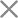HOTAPP下载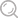# GRE备考：GRE数学重难点深度解析

GRE数学的考点主要是涵盖了国内高中以下的部分，整体上可以分为算术、代数、几何与数据分析资格章节，那么今天雷哥GRE分享的是由雷哥网老师带来的就GRE数学两大重难点进行的讲解。

①整数的奇偶性：一般是要求考生判断结果的奇偶性，主要掌握有关奇偶性计算的公式，奇数+奇数=偶数，偶数+偶数=偶数，奇数+偶数=奇数等等。

r and t are consecutive integers and p=r²+t。

Quantity A：(-1)^p

Quantity B：-1

A.Quantity A is greater.

B.Quantity B is greater.

C.The two quantities are equal.

D. The relationship cannot be determined from the information given.

【答案】C

【解析】r和t是连续整数，那么r和t当中一定是一个奇数一个偶数，r²与r本身的奇偶性是一致的，所以r²+t就是奇数+偶数，结果是奇数。因此(-1)^p=-1，QA=QB。

②因数与倍数：主要是考察对于因数倍数的判断，这部分会有很对题目跟质数相关，因数里面也有判断质因数的问题。

2600 has how many positive divisors?

A.6

B.12

C.18

D.24

E.48

【答案】D

【解析】求2600一共有多少正的因数，首先要将2600分解质因数，

2600=2³×5²×13，然后将每个质因数的指数都加1之后乘起来就可以，所以2600的正的因数个数就是(3+1)×(2+1)×(1+1)=24个。

③余数：这部分要求考生掌握余数相关的性质，做题主要多利用表达式，被除数=除数×商+余数，一般会要求求余数或者被除数。

If 2 is the remainder when m is divided by 5, what is the remainder when 3m is divided by 5?

A. 1

B. 2

C. 3

D. 4

E. 0

【答案】A

【解析】已知m除以5的余数是2，那么可以列表达式，假设m除以5的商是k，那么m=5k+2，由此3m=3(5k+2)=15k+6，3m÷5=(3k+1)……1，余数就是1.

①排列：从n个不同元素中，任取m(m≤n,m与n均为自然数)个元素按照一定的顺序排成一列，叫做从n个不同元素中取出m个元素的一个排列;所有排列的个数，就叫做从n个不同元素中取出m个元素的排列数，用符号 A(n,m)表示。

A(n,m)= n!/(n-m)! =n(n-1)(n-2)……(n-m+1)

How many positive integers less than 10,000 are such that the product of their digits is 210?

A. 24

B. 30

C. 48

D. 54

E. 72

【答案】D

【解析】要求小于10000并且每个数位上的数字的乘积是210的数字有多少个，首先可以把210分解，210=2×3×5×7，如果是个四位数，就可以是由2,3,5,7，或1,5,6,7构成的四位数;如果是三位数，就可以是5,6,7构成的三位数，而且数学之间是有顺序要求的，所以总共的排列数有多少，数字就有多少个。

②组合：从n个不同元素中，任取m(m≤n)个元素并成一组，叫做从n个不同元素中取出m个元素的一个组合;所有组合的个数，就叫做从n个不同元素中取出m个元素的组合数。用符号 C(n,m) 表示。

C(n,m)= n!/[(n-m)! *m!]=n(n-1)(n-2)……(n-m+1)/m!

C(n,m)=C(n,n-m),(其中n≥m)

How many positive integers can be expressed as a product of two or more of the prime numbers 5,7,11,and 13 if no>A.Eight

B.nine

C.Ten

D.Eleven

E.Twelve

【答案】D

【解析】要从5,7,11,13这几个质数当中选两个或者多个，求选出来的数字的乘积有多少个。首先从选两个或多个数字，可以分三种情况：2个数字，3个数字，4个数字。然后选出的数字顺序变了乘积也是不会变的，所以只需要求出组合数就行。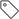​GRE数学 GRE备考 GRE考试 雷哥GRE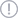报错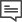网友评论
（评论数：0# 热门文章